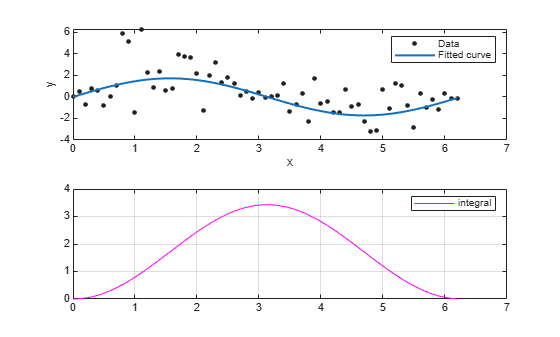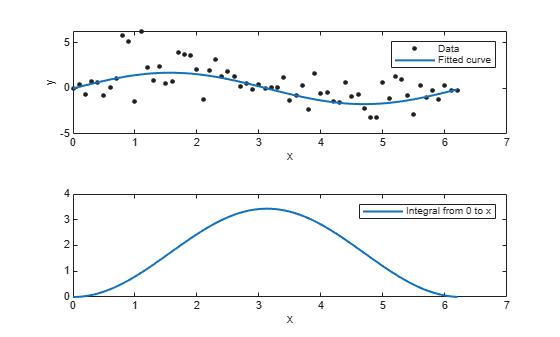# integrate

Integrate `cfit` object

## Syntax

``int = integrate(fun,x,x0)``

## Description

example

````int = integrate(fun,x,x0)` integrates the `cfit` object `fun` at the points specified by the vector `x`, starting from `x0`, and returns the result in `int`.```

## Examples

collapse all

Create a baseline sinusoidal signal.

```xdata = (0:.1:2*pi)'; y0 = sin(xdata);```

Add response-dependent Gaussian noise to the signal.

```noise = 2*y0.*randn(size(y0)); ydata = y0 + noise;```

Fit the noisy data with a custom sinusoidal model.

```f = fittype('a*sin(b*x)'); fit1 = fit(xdata,ydata,f,'StartPoint',[1 1]);```

Find the integral of the fit at the predictors.

`int = integrate(fit1,xdata,0);`

Plot the data, the fit, and the integral.

```subplot(2,1,1) plot(fit1,xdata,ydata) % cfit plot method subplot(2,1,2) plot(xdata,int,'m') % double plot method grid on legend('integral')```You can also compute integrals and plot them directly with the `cfit` `plot` method:

```figure plot(fit1,xdata,ydata,{'fit','integral'})```The `plot` method, however, does not return data on the integral.

## Input Arguments

collapse all

Function to integrate, specified as a `cfit` object.

Points at which to integrate the function, specified as a vector.

First point of integration, specified as a scalar.

## Output Arguments

collapse all

Result of the integration, returned as a vector of the same size of x.

## Version History

Introduced before R2006a